Next: A Basic Language Up: What is Constraint (Logic) Previous: Use Prolog as Host

Subsections

How Does a CLP System Work?

The reader might wonder how a CLP system actually works and solves equations. Equation solving in general might be radically different from the well-known methods for solving linear arithmetic equations. CLP programs set up equations just by expressing them; these equations, in an internally coded form, are communicated to an internal solver in which values for the variables are worked out. We will not be concerned with way these equations are encoded, but, for the sake of having more knowledge (which will help us in a future to write better CLP programs), we will become solvers of finite domain equations for a while.

Modeling the Problem

Suppose we have the precedence net (for example, for a project) and the task lengths1.3 in Figure 1.3.

Usual O.R. methods to find out critical tasks, the slacks in the tasks, the earliest finish time, etc. include the PERT and CPM algorithms. We will show how a simple, general finite domains algorithm performs the same task as those methods, and can even tackle more difficult problems within the same setting.Supposing that a hard limit for the length of the project is 10 time units, and that we choose each FD variable to represent the time in which the corresponding task can start, a model of the problem can be the following: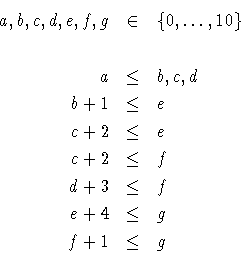The value of each variable (which is a set, initialized to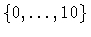) represents the moments in time the corresponding task can start. This equation cannot be solved using linear arithmetic methods, because the values of the variables are not real numbers, but rather sets of integers. Of course, it might reformulated in this particular, linear, case to use real numbers, but in general finite domains can always find a solution, because enumeration is possible, as we will see later.

Be a Solver

We will set up a tableau (Table 1.1) in which current domains for the variables will be stored at each moment. At the beginning, all variables will have the initial domain, and we will iterate using the following strategy:

• Choose one equation; analyze the values of the variables related by that equation.
• Sometimes the maximum / minimum values of the variables can be updated to make the equation hold. This causes the domain of the variable to be narrowed.
• Finish when no equation gives raise to a variable updating.

Table 1.1: Being a solver
 Variables and Domains Step a b c d e f g 0 0..10 0..10 0..10 0..10 0..10 0..10 0..10 1 0..9 1..10 2 0..8 2..10 3 2..10 4 0..7 3..10 5 2..6 6..10 6 3..9 7 0..7 8 0..5 9 0..4 10 0..6 11 0..4 Final domains 0..4 0..5 0..4 0..6 2..6 3..9 6..10

For example, in step 1, we have selected equation. Since previouslyand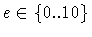, it is not difficult to deduce that b can be, at most, 9, and that e can be, at least, 1. So this step updates the domain of b and e to be, respectively,and. The rest of the steps perform similar operations, selecting other equations and refining values of variables until a fixpoint, in which no further changes can be made, is reached.

Although the general idea behind finite domain solver is as shown above, actual algorithms are much more complicated, and take into account issues like inequality constraints, global constraints, heuristics, enumeration, etc. More complex constraint solvers make a series of decisions (such as which equation is to be to chosen next) and, even if those do not affect correctness, performance depends heavily on them.

What are in this cases the differences with respect to classical methods, such as CPM? A central point is that this is just a particular application of finite domains, and not an algorithm dedicated to project scheduling. In fact, it gives more information than CPM, and can, using the same ideas, be used for more advanced tasks. For example, exact slacks of tasks in the critical path can be found just by setting g = 6 (which is just a way of writing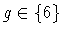, another equation similar to those we already have) and repeating the process. In fact, restart the solving from scratch is not necessary: as an example of the dynamic, incremental nature of CLP, we only need to add to our initial set of constraints the aforementioned equation, update the domain of g and then continue the process where it stopped before: more updates are now possible. The result will give us slacks for the variables and the start time for every task so that the project is finished in the shortest time possible.

Problem 1.1   Solve the problem with the added constraint that the final task must end at time 6.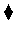Modeling other relationships without resorting to very different algorithms is also possible: for example,

• Two tasks do not depend on each other, but they cannot start at the same time: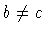.
• A resource r, of which there is a limited amount, is needed by two tasks b and d, and allocating more resource to one of these tasks speeds up its completion: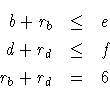Don't Be a Solver!

But the programmer using CLP tools does not need to build tableaus, keep track of communicating with the solver when a new constraint is added, or jump back to a point where a selection was previously done. CLP languages take care of all of these tasks by themselves, transparently to the programmer. Built-in solvers are provided for several constraint domains, FD being just one of them. Others, which we will talk about later, include linear equations, non-linear equations with intervals, boolean equations, etc.

In the next chapter we will have a look at a basic language, based on concepts taken from Logic Programming, and we will introduce the concepts of logical variables and backtracking. We will add constraint solving capabilities upon this simple language, and we will see how non-trivial problems are easy to express. We have chosen to use a logic programming basic language because, although some initial acquaintance with its peculiarities is needed, once this is mastered, the resulting language and syntax merges much better than other approaches with the idea of programming using constraints.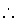Next: A Basic Language Up: What is Constraint (Logic) Previous: Use Prolog as Host
MCL
1998-12-03